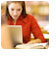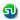### Logarithm, log question, Answers. Math Prep. Ascent MBA TANCET, XAT, CAT, PGSEM Preparation, Correspondence course

 Home TANCET Classes GMAT Coaching Online GMAT Course CAT Classes TANCET Study Material
 TANCET '18 Classroom ProgramWeekend and weekday classes for TANCET MBA @ Chennai. At Nungambakkam and Velachery.Other Courses TANCET Correspondence Course CAT Classes & Courses GMAT Classes Chennai Online GMAT Course GRE Classes Chennai CBSE Math Online Tuition SAT Classes Other Links Ascent TANCET Toppers Testimonials CAT, TANCET Questions Careers @ Ascent Contact Us +91 44 4500 8484 +91 96000 48484 ascent@ascenteducation.com Postal Address Facebook / Twitter / Blog / Videos
You are here: Home  »  CAT, XAT, TANCET Prep Questions »  Miscellaneous Topics »   Logarithm

# Quantitative Reasoning : Logarithms

## Question

If log102 = 0.3010, then find how many digits are contained in the number 256?
1. 15
2. 16
3. 17
4. 19

Choice (3). 17 digits

## Note:

To find the number of digits contained in the number, increment the characteristic (integral part) of the logarithmic value of the number by one.

Taking log of the given number, we get log10256 = 56* log102 = 56 * 0.3010 = 16.85.

In this case, the characteristic (integral part) = 16.

Therefore, number of digits contained in the number 256 is 16 + 1 = 17.

## CAT, XAT, TANCET Practice Questions and Answers : Listed Topicwise

 Number Theory Permutation Combination Probability Inequalities Geometry Mensuration Trigonometry Coordinate Geometry Percentages Profit Loss Ratio Proportion Mixtures Alligation Speed Time Distance Pipes Cisterns Interest Races Average, Mean AP, GP, HP Set Theory Clocks Calendars Algebra Function English Grammar General Awareness Data sufficiency TANCET Papers XAT PapersAdd to del.icio.usStumble Itdigg this